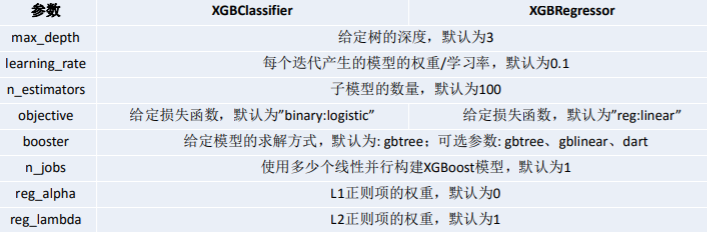# XGBoost 重要参数(调参使用)

## XGBoost 的重要参数XGBoost的参数一共分为三类：

1. 通用参数：宏观函数控制。

2. Booster参数：控制每一步的booster(tree/regression)。booster参数一般可以调控模型的效果和计算代价。我们所说的调参，很这是大程度上都是在调整booster参数。

3. 学习目标参数：控制训练目标的表现。我们对于问题的划分主要体现在学习目标参数上。比如我们要做分类还是回归，做二分类还是多分类，这都是目标参数所提供的。

### 通用参数

1. booster：我们有两种参数选择，gbtreegblinear。gbtree是采用树的结构来运行数据，而gblinear是基于线性模型。

2. silent：静默模式，为1时模型运行不输出。

3. nthread: 使用线程数，一般我们设置成-1,使用所有线程。如果有需要，我们设置成多少就是用多少线程。

### Booster参数

1. n_estimator: 也作num_boosting_rounds

这是生成的最大树的数目，也是最大的迭代次数。

2. learning_rate: 有时也叫作eta，系统默认值为0.3,。

每一步迭代的步长，很重要。太大了运行准确率不高，太小了运行速度慢。我们一般使用比默认值小一点，0.1左右就很好。

3. gamma：系统默认为0,我们也常用0

在节点分裂时，只有分裂后损失函数的值下降了，才会分裂这个节点。gamma指定了节点分裂所需的最小损失函数下降值。 这个参数的值越大，算法越保守。因为gamma值越大的时候，损失函数下降更多才可以分裂节点。所以树生成的时候更不容易分裂节点。范围: [0,∞]

4. subsample：系统默认为1

这个参数控制对于每棵树，随机采样的比例。减小这个参数的值，算法会更加保守，避免过拟合。但是，如果这个值设置得过小，它可能会导致欠拟合。 典型值：0.5-10.5代表平均采样，防止过拟合. 范围: (0,1]注意不可取0

5. colsample_bytree：系统默认值为1。我们一般设置成0.8左右。

用来控制每棵随机采样的列数的占比(每一列是一个特征)。 典型值：0.5-1范围: (0,1]

6. colsample_bylevel：默认为1,我们也设置为1.

这个就相比于前一个更加细致了，它指的是每棵树每次节点分裂的时候列采样的比例

7. max_depth： 系统默认值为6

我们常用3-10之间的数字。这个值为树的最大深度。这个值是用来控制过拟合的。max_depth越大，模型学习的更加具体。设置为0代表没有限制，范围: [0,∞]

8. max_delta_step：默认0,我们常用0.

这个参数限制了每棵树权重改变的最大步长，如果这个参数的值为0,则意味着没有约束。如果他被赋予了某一个正值，则是这个算法更加保守。通常，这个参数我们不需要设置，但是当个类别的样本极不平衡的时候，这个参数对逻辑回归优化器是很有帮助的。

9. lambda:也称reg_lambda,默认值为0

权重的L2正则化项。(和Ridge regression类似)。这个参数是用来控制XGBoost的正则化部分的。这个参数在减少过拟合上很有帮助。

10. alpha:也称reg_alpha默认为0,

权重的L1正则化项。(和Lasso regression类似)。 可以应用在很高维度的情况下，使得算法的速度更快。

11. scale_pos_weight：默认为1

在各类别样本十分不平衡时，把这个参数设定为一个正值，可以使算法更快收敛。通常可以将其设置为负样本的数目与正样本数目的比值。

### 学习目标参数

#### objective [缺省值=reg:linear]

• reg:linear– 线性回归

• reg:logistic – 逻辑回归

• binary:logistic – 二分类逻辑回归，输出为概率

• binary:logitraw – 二分类逻辑回归，输出的结果为wTx

• count:poisson – 计数问题的poisson回归，输出结果为poisson分布。在poisson回归中，max_delta_step的缺省值为0.7 (used to safeguard optimization)

• multi:softmax – 设置 XGBoost 使用softmax目标函数做多分类，需要设置参数num_class（类别个数）

• multi:softprob – 如同softmax，但是输出结果为ndata*nclass的向量，其中的值是每个数据分为每个类的概率。

#### eval_metric [缺省值=通过目标函数选择]

• rmse: 均方根误差

• mae: 平均绝对值误差

• logloss: negative log-likelihood

• error: 二分类错误率。其值通过错误分类数目与全部分类数目比值得到。对于预测，预测值大于0.5被认为是正类，其它归为负类。 error@t: 不同的划分阈值可以通过 ‘t’进行设置

• merror: 多分类错误率，计算公式为(wrong cases)/(all cases)

• mlogloss: 多分类log损失

• auc: 曲线下的面积

• ndcg: Normalized Discounted Cumulative Gain

• map: 平均正确率

## 两种基本的实例

from numpy import loadtxt
from xgboost import XGBClassifier
from sklearn.model_selection import train_test_split
from sklearn.metrics import accuracy_score
​
# 导入数据
# split data into X and y
X = dataset[:, 0:8]
Y = dataset[:, 8]
# split data into train and test sets
seed = 7
test_size = 0.33
X_train, X_test, y_train, y_test = train_test_split(X, Y, test_size=test_size, random_state=seed)
# 设置参数
model = XGBClassifier(max_depth=15,
learning_rate=0.1,
n_estimators=2000,
min_child_weight=5,
max_delta_step=0,
subsample=0.8,
colsample_bytree=0.7,
reg_alpha=0,
reg_lambda=0.4,
scale_pos_weight=0.8,
silent=True,
objective='binary:logistic',
missing=None,
eval_metric='auc',
seed=1440,
gamma=0)
model.fit(X_train, y_train)
# 进行预测
y_pred = model.predict(X_test)
predictions = [round(value) for value in y_pred]
# 查看准确率
accuracy = accuracy_score(y_test, predictions)
print("Accuracy: %.2f%%" % (accuracy * 100.0))

import xgboost as xgb
from sklearn.model_selection import train_test_split
from sklearn.metrics import roc_auc_score
# 二分类解决乳腺癌
x = cancer.data
y = cancer.target
train_x, valid_x, train_y, valid_y = train_test_split(x, y, test_size=0.333, random_state=0)  # 分训练集和验证集
# 这里不需要Dmatrix
​
xlf = xgb.XGBClassifier(max_depth=10,
learning_rate=0.01,
n_estimators=2000,
silent=True,
objective='binary:logistic',
gamma=0,
min_child_weight=1,
max_delta_step=0,
subsample=0.85,
colsample_bytree=0.7,
colsample_bylevel=1,
reg_alpha=0,
reg_lambda=1,
scale_pos_weight=1,
seed=1440,
missing=None)
xlf.fit(train_x, train_y, eval_metric='error', verbose=True, eval_set=[(valid_x, valid_y)], early_stopping_rounds=30)
# 这个verbose主要是调节系统输出的，如果设置成10,便是每迭代10次就有输出。
# 注意我们这里eval_metric=‘error’便是准确率。这里面并没有accuracy命名的函数，网上大多例子为auc，我这里特意放了个error。
y_pred = xlf.predict(valid_x, ntree_limit=xlf.best_ntree_limit)
auc_score = roc_auc_score(valid_y, y_pred)
y_pred = xlf.predict(valid_x, ntree_limit=xlf.best_ntree_limit)
# xgboost没有直接使用效果最好的树作为模型的机制，这里采用最大树深限制的方法，目的是获取刚刚early_stopping效果最好的，实测性能可以
auc_score = roc_auc_score(valid_y, y_pred)  # 算一下预测结果的roc值

## GridSearch 简介

import xgboost as xgb
from sklearn.model_selection import train_test_split
from sklearn.model_selection import GridSearchCV
​
x = cancer.data[:50]
y = cancer.target[:50]
train_x, valid_x, train_y, valid_y = train_test_split(x, y, test_size=0.333, random_state=0)  # 分训练集和验证集
# 这里不需要Dmatrix
​
parameters = {
'max_depth': [5, 10, 15, 20, 25],
'learning_rate': [0.01, 0.02, 0.05, 0.1, 0.15],
'n_estimators': [50, 100, 200, 300, 500],
'min_child_weight': [0, 2, 5, 10, 20],
'max_delta_step': [0, 0.2, 0.6, 1, 2],
'subsample': [0.6, 0.7, 0.8, 0.85, 0.95],
'colsample_bytree': [0.5, 0.6, 0.7, 0.8, 0.9],
'reg_alpha': [0, 0.25, 0.5, 0.75, 1],
'reg_lambda': [0.2, 0.4, 0.6, 0.8, 1],
'scale_pos_weight': [0.2, 0.4, 0.6, 0.8, 1]
​
}
​
xlf = xgb.XGBClassifier(max_depth=10,
learning_rate=0.01,
n_estimators=2000,
silent=True,
objective='binary:logistic',
gamma=0,
min_child_weight=1,
max_delta_step=0,
subsample=0.85,
colsample_bytree=0.7,
colsample_bylevel=1,
reg_alpha=0,
reg_lambda=1,
scale_pos_weight=1,
seed=1440,
missing=None)
​
# 有了gridsearch我们便不需要fit函数
gsearch = GridSearchCV(xlf, param_grid=parameters, scoring='accuracy', cv=3)
gsearch.fit(train_x, train_y)
​
print("Best score: %0.3f" % gsearch.best_score_)
print("Best parameters set:")
best_parameters = gsearch.best_estimator_.get_params()
for param_name in sorted(parameters.keys()):
print("\t%s: %r" % (param_name, best_parameters[param_name]))
#极其耗费时间,电脑没执行完

Possible inputs for cv are:

• None, to use the default 3-fold cross-validation,

• integer, to specify the number of folds.

• An object to be used as a cross-validation generator.

• An iterable yielding train/test splits.

cv的可能输入包括:

• None，使用默认的3倍交叉验证，

• 整数，用来指定折叠的次数。

• 用作交叉验证生成器的对象。

• 一个可迭代的训练/测试序列进行分割。

posted @ 2019-08-30 17:04  Timcode  阅读(...)  评论(...编辑  收藏